# Cyclical SGLD in Blackjax

### Cyclical schedule

from typing import NamedTuple

class ScheduleState(NamedTuple):
step_size: float
do_sample: bool

def build_schedule(
num_training_steps,
num_cycles=4,
initial_step_size=1e-3,
exploration_ratio=0.25,
):
cycle_length = num_training_steps // num_cycles

def schedule_fn(step_id):
do_sample = False
if ((step_id % cycle_length)/cycle_length) >= exploration_ratio:
do_sample = True

cos_out = jnp.cos(jnp.pi * (step_id % cycle_length) / cycle_length) + 1
step_size = 0.5 * cos_out * initial_step_size

return ScheduleState(step_size, do_sample)

return schedule_fn


Let us visualize the schedule for 200k training steps divided in 4 cycles. At each cycle 1/4th of the steps are dedicated to exploration.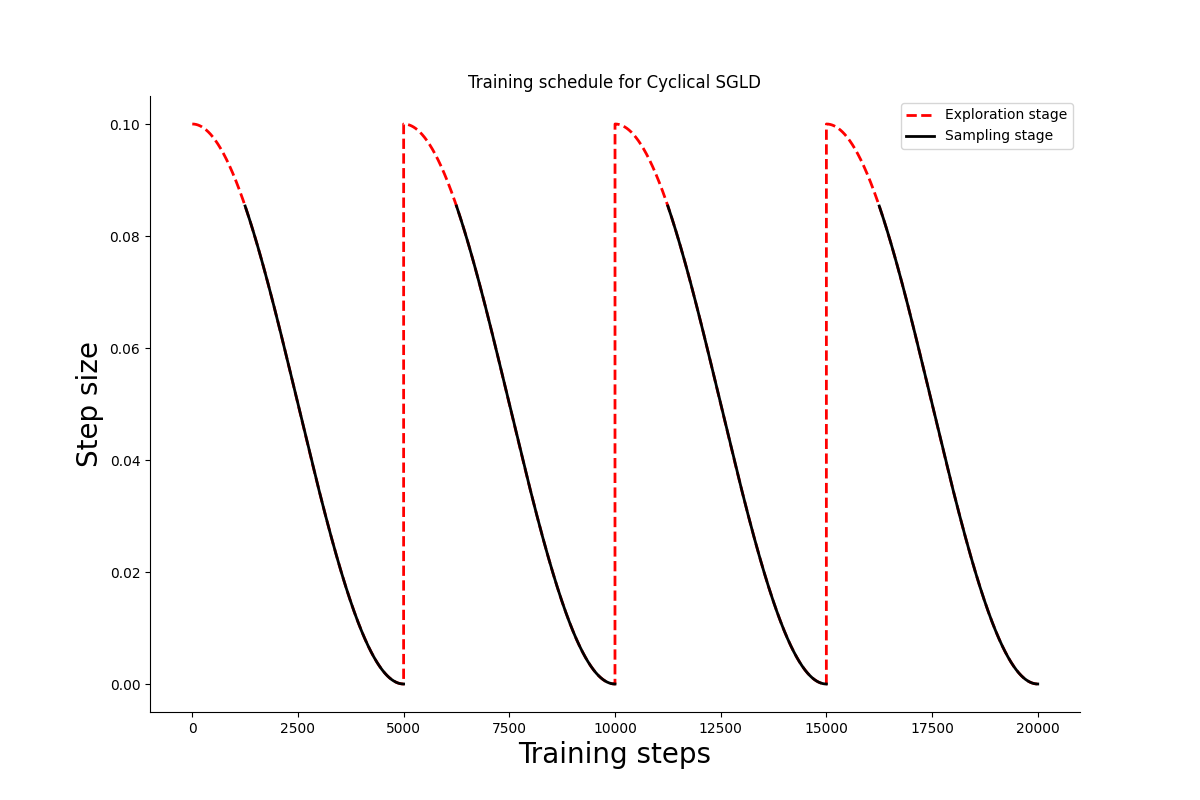### Cyclical SGLD step

We will reproduce one of the paper's example, sampling from an array of 25 gaussians.

import itertools

import jax
import jax.scipy as jsp
import jax.numpy as jnp

lmbda = 1/25
positions = [-4, -2, 0, 2, 4]
mu = jnp.array([list(prod) for prod in itertools.product(positions, positions)])
sigma = 0.03 * jnp.eye(2)

def logprob_fn(x, *_):
return lmbda * jsp.special.logsumexp(
jax.scipy.stats.multivariate_normal.logpdf(x, mu, sigma)
)

def sample_fn(rng_key):
choose_key, sample_key = jax.random.split(rng_key)
samples = jax.random.multivariate_normal(sample_key, mu, sigma)
return jax.random.choice(choose_key, samples)


Let's plot the model's density; we will need the plot later to evaluate the sampler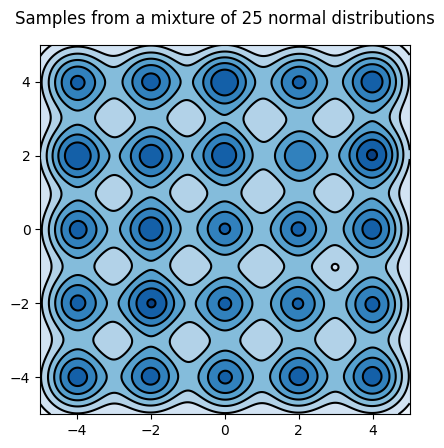### Sample from the mixture of gaussians

The sampling kernel must be able to alternate between sampling and optimization periods that are determined by the scheduler.

from typing import NamedTuple

import blackjax
import optax

from blackjax.types import PyTree
from optax._src.base import OptState

class CyclicalSGMCMCState(NamedTuple):
"""State of the Cyclical SGMCMC sampler."""
position: PyTree
opt_state: OptState

sgd = optax.sgd(1.)

def init_fn(position):
"""Initialize Cyclical SGLD's state."""
opt_state = sgd.init(position)
return CyclicalSGMCMCState(position, opt_state)

def step_fn(
rng_key,
schedule_state: ScheduleState,
state: CyclicalSGMCMCState,
minibatch: PyTree
):
"""Cyclical SGLD kernel.

TODO: Organize the inputs to match the SGLD API better.

rng_key
Key for JAX's pseudo-random number generator.
schedule_state
The current state of the scheduler. Indicates whether the kernel
should be sampling or optimizing, and the current step size.
state
The current state of the Cyclical SGLD sampler.
minibatch
Not used in the mixture example, but this is where you would pass
batches of data in any real application.

"""

def step_with_sgld(current_state):
rng_key, state, minibatch, step_size = current_state
new_position = sgld(rng_key, state.position, minibatch, step_size)
return CyclicalSGMCMCState(new_position, state.opt_state)

def step_with_sgd(current_state):
_, state, minibatch, step_size = current_state
return CyclicalSGMCMCState(new_position, new_opt_state)

new_state = jax.lax.cond(
schedule_state.do_sample,
step_with_sgld,
step_with_sgd,
(rng_key, state, minibatch, schedule_state.step_size)
)

return new_state

return init_fn, step_fn


### SGLD

import jax
from fastprogress import progress_bar

# 50k iterations
num_training_steps = 50000
schedule_fn = lambda k: 0.05 * k ** (-0.55)
# TODO: There is no need to pre-compute the schedule
schedule = [schedule_fn(i) for i in range(1, num_training_steps+1)]

rng_key = jax.random.PRNGKey(3)
init_position = -10 + 20 * jax.random.uniform(rng_key, shape=(2,))

position = init_position
sgld_samples = []
for i in progress_bar(range(num_training_steps)):
_, rng_key = jax.random.split(rng_key)
position = jax.jit(sgld)(rng_key, position, 0, schedule[i])
sgld_samples.append(position)


Let's plot the trajectory: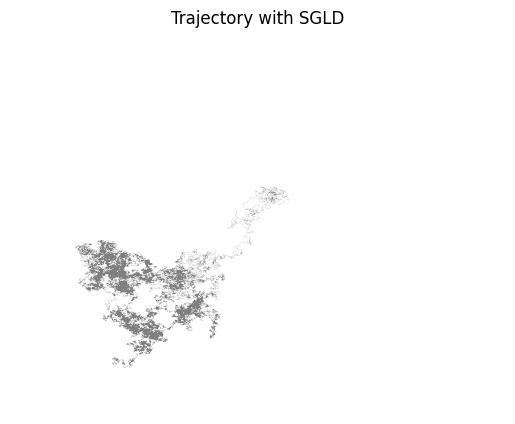### Cyclical SGLD

Now let's sample using Cyclical SGLD.

import jax
from fastprogress import progress_bar

# 50k iterations
# M = 30
# initial step size = 0.09
# ratio exploration = 1/4
num_training_steps = 50000
schedule_fn = build_schedule(num_training_steps, 30, 0.09, 0.25)
# TODO: There is no need to pre-compute the schedule
schedule = [schedule_fn(i) for i in range(num_training_steps)]

rng_key = jax.random.PRNGKey(3)
init_position = -10 + 20 * jax.random.uniform(rng_key, shape=(2,))
init_state = init(init_position)

state = init_state
cyclical_samples = []
for i in progress_bar(range(num_training_steps)):
_, rng_key = jax.random.split(rng_key)
state = jax.jit(step)(rng_key, schedule[i], state, 0)
if schedule[i].do_sample:
cyclical_samples.append(state.position)


It looks from the trajectory that the distribution is better explored: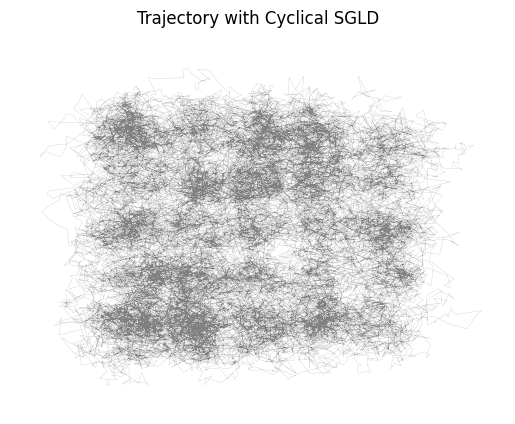Let's look at the distribution: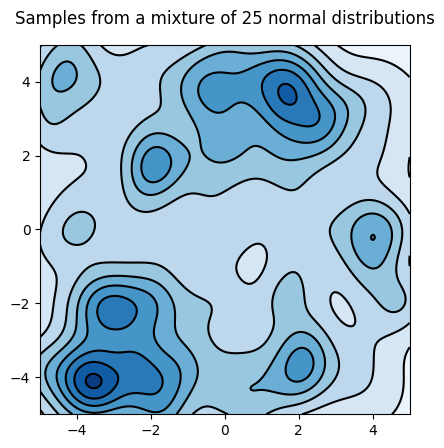## What's next

• As Adrien Corenflos noted, Scott's rule for KDE assumes that the total number of points is the sample size, so is not fit for MCMC samples. We should instead pass the bandwidth manually $$n_{eff}^{(-1/(d+4))}$$ with $$d=2$$; It should capture more modes.
• Compute the paper's Mode-coverage metric: when the number of samples falling within the radius $$r$$ of a mode center is larger than a number $$N$$ when we say the mode is covered;
• Use on a "real" problem: CIFAR-100 with Resnet18 for instance;